# The Landweber exact-functor theorem

This post assumes familiarity with formal group laws, the definition of exact sequences, the motivation of the Landweber-Ravenel-Stong construction, that the exactness axioms are one of the generalized Eilenberg-Steenrod axioms, and the fact that formal group laws over $$R$$ are represented by maps from the Lazard ring to $$R$$.

Recall the the Landweber-Ravenel-Stong Construction: $$MU^(X) \otimes_{L} R \simeq E^(X)$$, where $$MU^* \simeq L$$ and $$R \simeq E^*(pt)$$.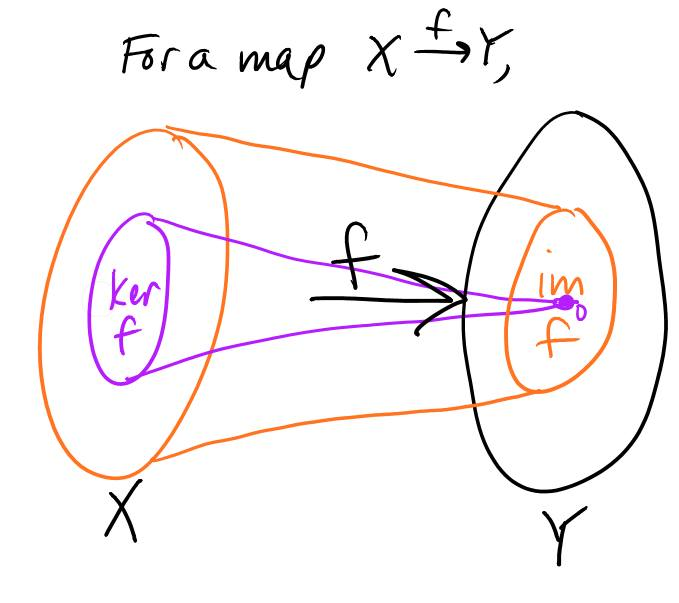We know that in general, tensoring with abelian groups does not preserve exact sequences (e.g., applying $$-\otimes{\mathbb{Z}} \mathbb{Z}/2$$ to $$0 \to \mathbb{Z} \xrightarrow{\times p} \mathbb{Z} \to \mathbb{Z}/p \to 0$$)._

So, when does the functor $$-\otimes_L R: MU^(X) \to E^(X)$$ preserve exact sequences?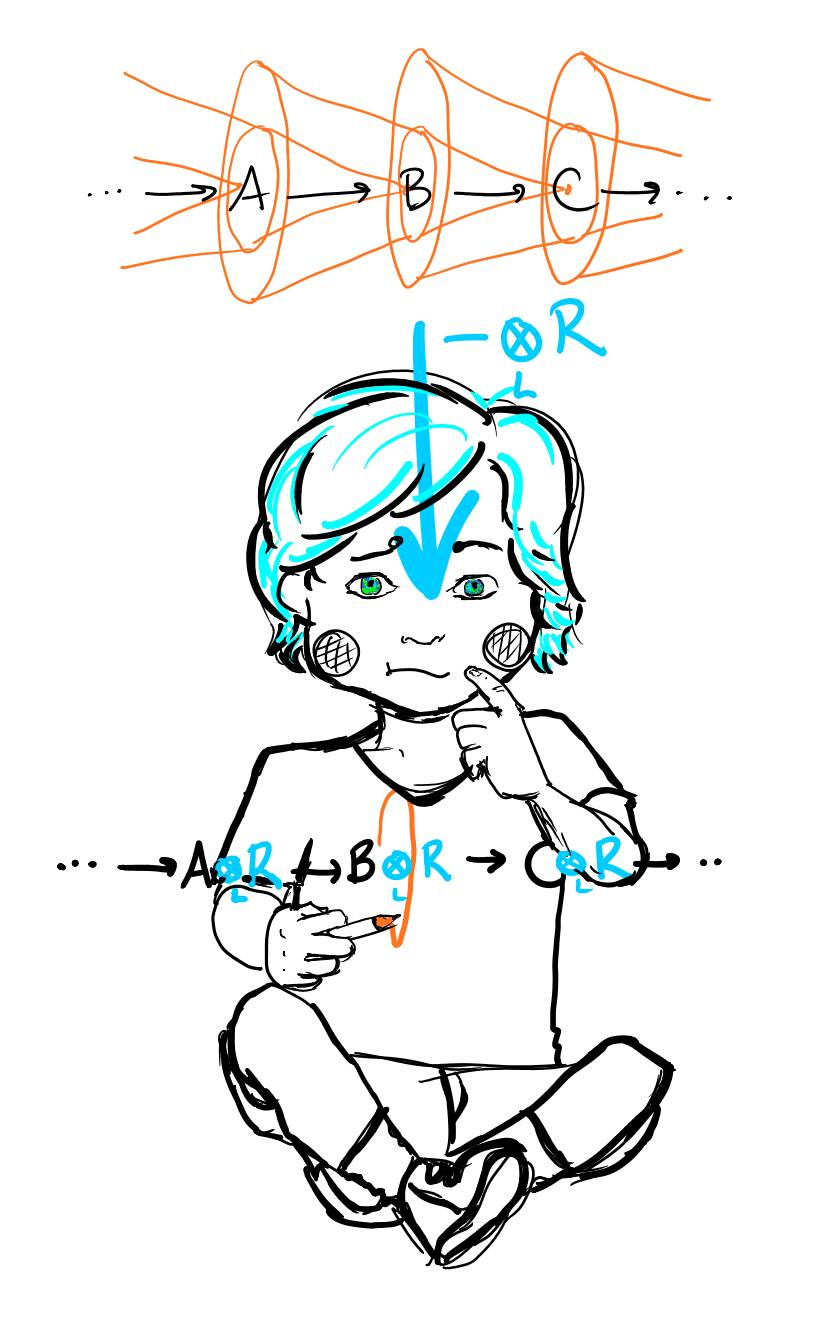An object M in an abelian tensor category $$C$$ is ‘flat’ if for all $$X \in Obj(C)$$, the functor $$X \to X \otimes M$$ preserves exact sequences.

Because arbitrary $$MU_$$-modules do not occur as the $$MU$$-homology of spaces, the requirement of flatness over all of $$MU_$$ can be relaxed.

It is Landweber-exact if it preserves exactness when applied to things in the range of the homology theory, though it doesn’t have to preserve exactness on things that aren’t in the range of the homology theory. (summarization by Alex Mennen)

Sidenote: All we require is that the map $$\text{Spec }R \to M{FG}$$ is flat. Why to $$M_{FG}$$ and not to $$M_{FGL}$$? Every (complex orientable) cohomology theory corresponds to a formal group; picking a complex orientation corresponds to a choice of coordinate of our formal group law._

I say $$MU$$ instead of $$MU^$$ for technical reasons, namely, colimits don’t behave nicely in cohomology. But I don’t understand the implications of that well enough to talk about it coherently._

You may ask why we’re only checking for exactness! Since we’re dealing only with CW-complexes: the excision axiom automatically holds, the homotopy equivalence axiom automatically holds because we’re applying a functor to $$MU$$ (functors preserve isomorphisms), and additivity always holds. All we really need to check is exactness.

Flatness and torsion are intimately related. It’s a theorem of Lazard that an object is flat iff it’s a filtered colimit of free modules.

Let’s say we have a map from the Lazard ring $$L \xrightarrow{F} R$$ representing our formal group law $$F$$. What is this exactness condition, precisely?

Let’s back up a bit and look at what it might mean for a formal group law to be “flat.”

We wish to “add” a point to itself via the formal group law $$p$$ times and look at its general form (this is called the $$p$$-series of our formal group law). This allows us to detect stuff like points of order $$2$$ in elliptic curves, that is, the points that when added to themselves give us the origin. Note that $$p$$ is a prime in $$\mathbb{Z}$$, not necessarily a prime in $$R$$.

In general, we can talk about adding a point $$x$$ to itself $$n$$ times using the following recursive definition: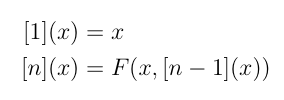It doesn’t seem like it at first glance, p-series, or “multiplication by p” map, is EXTREMELY IMPORTANT — it allows us to find periodic phenomena. Let’s look at the map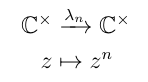The kernel of $$\lambda_n$$ will be the roots of unity in $$C$$.

Similarly, let’s look at the group $$G$$.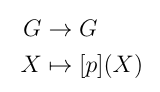The kernel of this map will be the points in $$G$$ with an order that divides $$p$$. If $$p$$ is a prime, it’s just the points of order $$p$$.

Examples of $$p$$-series: Additive formal group law $$F(x, y) = x + y$$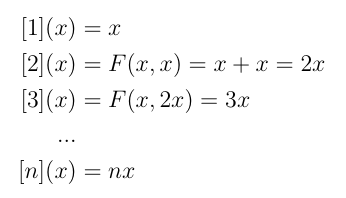Multiplicative formal group law $$F(x, y) = x + y + cxy$$, where $$c = 1$$ we’ll be working with $$c = -1$$ at some point later in the post, sorry for the inconsistency!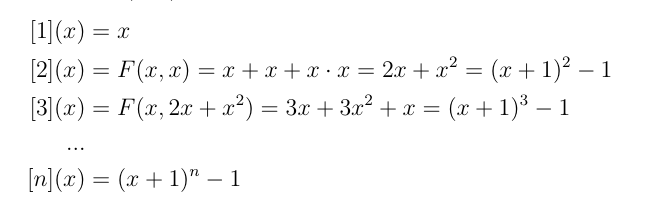The $$p$$-series of $$F$$ will always be of the form:

$$p = px + … + v_1x^{p^1} + … + v_nx^{p^n} + …$$

where $$v_k$$ is simply the name we give the coefficient of the expression $$x^{p^k}$$.

We’re interested in the tuple of coefficients relevant to the powers of $$p$$, that is $$(p, v_1, …, v_k, …)$$. Let’s mod out the $$p$$-series by $$p$$, then by $$v_1$$, etc. until we get to $$0$$. We are trying to check that $$v_n$$ acts injectively in $$R/(v_0, …, v_{n-1})$$ for all $$n$$.

Note that $$p$$ is a prime in $$\mathbb{Z}$$ and $$v_1, …, v_n \in$$ the image of $$MU^*$$ \in $$R$$. The condition of “regular” wards away zero divisors because they are nasty.

For example, our tuple for the multiplicative formal group law is $$(p, 1)$$, since $$v_1 = 1$$, and the rest of the coefficients are 0, so we have: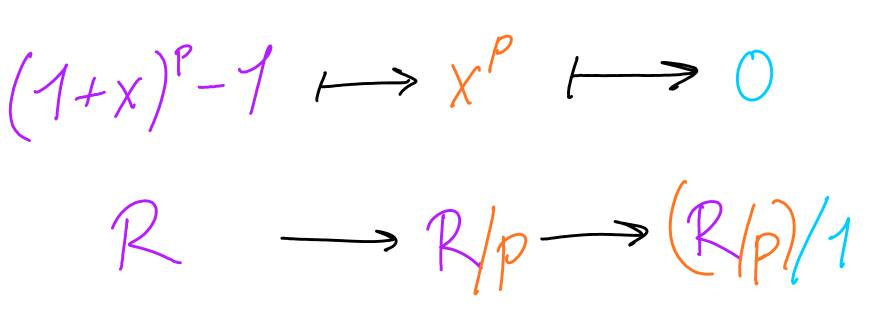Tada! It’s Landweber exact so you can bet your muffins that $$MU^(X) \otimes_L \mathbb{Z}$$ is a cohomology theory, in fact, it’s iso to $$K^(X)$$.

That’s a lot to take in, I know, so let’s back up a bit and examine the map $$Vect^1(X) \xrightarrow{c_1} K^2(X; \mathbb{Z})$$ where the group operators are the tensor product of line bundles and the tensor product of virtual line bundles.

Note that the dimensions multiply when you do the tensor product, so $$L_1 \otimes L_2$$ is still a line bundle.

Let’s say that $$c_1(L) = 1-L$$, then we’d expect $$c_1(L_1 \otimes L_2) = 1 - L_1 \otimes L_2$$. So, how do we express $$c_1(L_1 \otimes L_2)$$ in terms of a formal group law $$F(x,y)$$ where $$x = c_1(L_1)$$, and $$y = c_1(L_2))$$?

In other words, using the slightly clearer notation $$F(x,y) \equiv x +_F y$$…for what $$F$$ does $$x +_F y = 1-xy$$?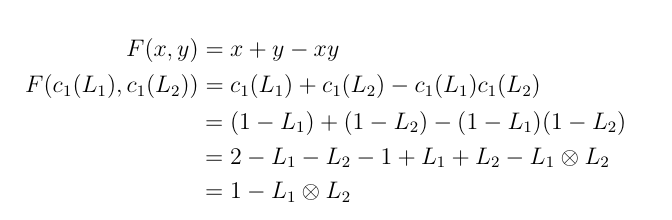Lost in the algebra? Worry not, I have something that may appeal to your geometric taste: the striking perspective of Morava, in Forms of K-theory.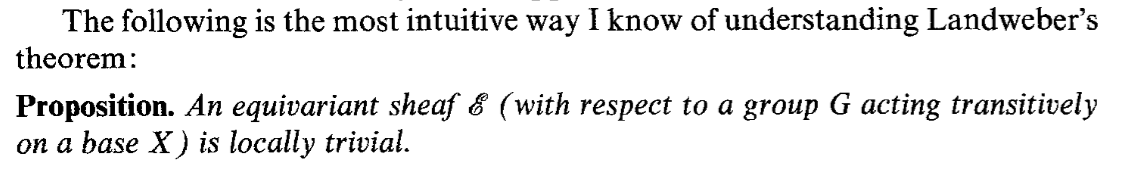We can use the properties of the parameterized space below (the set of W-valued genera with the Zariski topology, a point of this space is a formal group law of height 1 over W) to prove things about the stalks above (K-theories associated to those genera). It’s a pretty beautiful proof method, once we establish the following two claims…

1. moduli space below has a transitive group action, f $$F(X, Y) \mapsto f^{-1}F(f(X), F(Y))$$
2. we know at least one of the stalks is exact (the topological K-theory we know and love).

…we know that the rest of the stalks are exact.

Now that I’ve gotten you riled up, let’s back away from $$K$$-theory.

I wrote this post because I was really excited about the following: What if we wish to look at the cohomology theory associated to a supersingular elliptic curve in characteristic $$p$$?

We can’t have torsion in the Landweber-Stong-Ravenel construction, however we can consider the elliptic curve over p-adic completion of $$\mathbb{Z}$$, and adjoin $$v_1$$, such that $$(\mathbb{Z}_p[[v_1]]/p)/v_1 = \mathbb{Z}/p$$.

WOO! Actually, this isn’t too surprising. Recall that the p-adics are the limit of $$\mathbb{Z}/p^n$$, thus by definition it comes with maps to all the guys in the limit.

#### Afternote:

It might be tempting to think that all even periodic cohomology theories (that is, all cohomology theories $$E$$ satisfying $$E^{n}() \otimes_{E^()} E^2() \simeq E^{n+2}()$$, and $$E^n() = 0$$ if n is odd) are associated to the multiplicative formal group law. This is not the case, for example, elliptic cohomology is even periodic (note that even periodic $$\subset$$ complex orientable).

Elliptic cohomology is not naturally even periodic. For a given cohomology theory $$E$$, even periodicity comes when we must force an ungraded ring into the world of graded rings. One way of doing this is the following:

$$E^n(pt) = \begin{cases} R & \mbox{if } n \mbox{ is even} \ 0 & \mbox{if } n \mbox{ is odd}. \end{cases} \pmod{2}$$

When we look at $$E^(pt)$$ as a whole, instead of just $$E^n(pt)$$ individually, we see that $$E^(pt) \simeq R[[v_1, v_1^{-1}]]$$ is an iso of graded rings.)

I’d also like to mention something slightly more obvious (than the trick with getting supersingular curves in char p through the Landweber-exactness condition) but still awesome:

Tensoring with $$\mathbb{Q}$$ gets rid of torsion. Recall that every formal group law over $$\mathbb{Q}$$ is isomorphic to the additive formal group law. The difference between cohomology theories arises due to torsion! $$E \otimes \mathbb{Q}$$ simply gives us singular cohomology with some coefficient ring!

This is true more generally: rational spectra (i.e., all the homotopy groups are $$\mathbb{Q}$$ - vector spaces) are always determined by their homotopy groups. More precisely: there is a functor from rational spectra to graded $$\mathbb{Q}$$ - vector spaces given by taking homotopy groups, and it’s an equivalence. I’m not yet sure why this is.

I’ve read that an elliptic curve formal group law must be of either height 0, 1, or 2 (1 if singular, 2 if super singular). Why can’t it be of higher height?

Thank you to Akhil Mathew for kindly answering my questions on Landweber-exactness, Achim Krause for talking with me about rational spectra, and the lovely people of Math Overflow for answering my question on Landweber-exactness.

Written on April 11, 2015